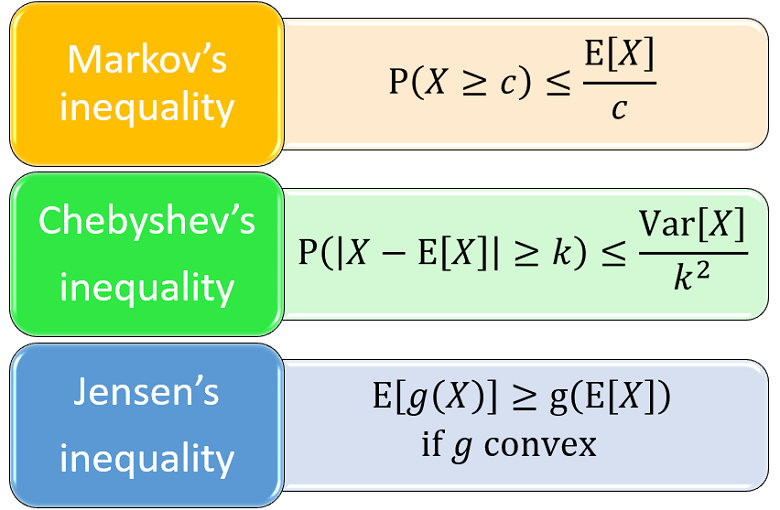StatLect

# Chebyshev's inequality

Chebyshev's inequality is a probabilistic inequality. It provides an upper bound to the probability that the absolute deviation of a random variable from its mean will exceed a given threshold.## Statement

The following is a formal statement.

Proposition Letbe a random variable having finite meanand finite variance. Let(i.e.,is a strictly positive real number). Then, the following inequality, called Chebyshev's inequality, holds:The proof is a straightforward application of Markov's inequality.

Proof

Sinceis a positive random variable, we can apply Markov's inequality to it: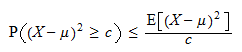By setting, we obtain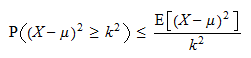Butif and only if, so we can write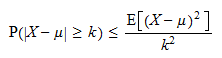Furthermore, by the very definition of variance,Therefore,## Example

Suppose that we extract an individual at random from a population whose members have an average income of \$40,000, with a standard deviation of \$20,000.

What is the probability of extracting an individual whose income is either less than \$10,000 or greater than \$70,000?

In the absence of more information about the distribution of income, we cannot compute this probability exactly. However, we can use Chebyshev's inequality to compute an upper bound to it.

Ifdenotes income, thenis less than \$10,000 or greater than \$70,000 if and only ifwhereand.

The probability that this happens is: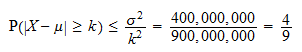Therefore, the probability of extracting an individual outside the income range \$10,000-\$70,000 is less than.

## Important applications

Chebyshev's inequality has many applications, but the most important one is probably the proof of a fundamental result in statistics, the so-called Chebyshev's Weak Law of Large Numbers.

## Solved exercises

Below you can find some exercises with explained solutions.

### Exercise 1

Letbe a random variable such thatFind a lower bound to its variance.

Solution

The lower bound can be derived thanks to Chebyshev's inequality:where: in stepwe have used the inequality; in stepwe have used the fact that; inwe have used the formula for the probability of a complement; in stepwe have used the monotonicity of probability: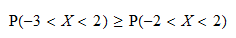Thus, the lower bound is### Exercise 2

Letbe a random variable such thatFind an upper bound to the probabilitySolution

We can solve this problem as follows: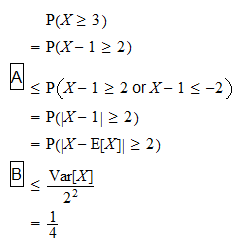where: in stepwe have used the monotonicity of probability; in stepwe have used Chebyshev's inequality.

## Other inequalities

If you like this page, StatLect has other pages on probabilistic inequalities: